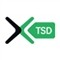1177

Hello,

Yes it is the frametime which I chose to test in first .

I also put in comparison the version of origin : .mql

with 4 majors paires.14401177

Yes I use it in demonstration but in account reel, my broker uses MT3 :cool:1177

Hello

I am not a programmer,you understood it well by reading my posts, however I am curious and by reading the EA with Metaeditor, I saw that:

extern int StartHour = 8;

extern int EndHour = 20;

does that want to say that this EA works only of 8h00 with 20h00 solely and not the night?

Thx1440

yes ... this trader not works in night11

Hello Forexts j don't know well the language for program with MQL language and j see the code program of "Volume_trader_v2";

j think that the volume is very important for trading operation.

In the Expert the condition for entry (Long || Short) is true || false if the (volumevolume) and this control is important.

J ask you if you can write the expert but the control for entry is moving average of volume for example three period is ">" of ten period and if this condition is true look if the close of baris ">" of close of bar  this is condition true for buy order .

Reverse condition for sell order.

Important insert the trailing stop and stop loss for example 10 for trailing and 15 for stop loss.

thank byeFiles:11

Hello Forexts j don't know well the language for program with MQL language and j see the code program of "Volume_trader_v2";

j think that the volume is very important for trading operation.

In the Expert the condition for entry (Long || Short) is true || false if the (volumevolume) and this control is important.

J ask you if you can write the expert but the control for entry is moving average of volume for example three period is ">" of ten period and if this condition is true look if the close of baris ">" of close of bar  this is condition true for buy order .

Reverse condition for sell order.

Important insert the trailing stop and stop loss for example 10 for trailing and 15 for stop loss.

See my algoritm if is correct please.

// Developed by www.forex-tsd.com

// Idea from John Taylor

// v.2.0

#include

#define MySuperMagic 111020051110

extern int StartHour = 8;

extern int EndHour = 20;

extern double Lots = 0.1;

int volume_medio,volume_attuale;

double LastBarChecked;

int init()

{

//----

LastBarChecked = Time;

//----

return(0);

}

//+------------------------------------------------------------------+

//| expert deinitialization function |

//+------------------------------------------------------------------+

int deinit()

{

return(0);

}

//| expert start function |

//+------------------------------------------------------------------+

int start()

{

string cm = "Volume ";

if (Period() == 1) cm = cm + "1M";

if (Period() == 5) cm = cm + "5M";

if (Period() == 15) cm = cm + "15M";

if (Period() == 30) cm = cm + "30M";

if (Period() == 60) cm = cm + "1H";

if (Period() == 240) cm = cm + "4H";

if (Period() == 1440) cm = cm + "1D";

if (Period() == 10080) cm = cm + "1W";

if (Period() == 43200) cm = cm + "1M";

cm = cm + " - ";

cm = cm + TimeToStr(CurTime(),TIME_DATE|TIME_SECONDS);

int EAMagic = MySuperMagic + Period();

//------------------------------------------------------------------------------------------------

bool doShort = false;

bool doLong = false;

//------------------------------------------------------------------------------------------------

bool long = false;

bool short=false;

//------------------------------------------------------------------------------------------------

volume_medio=iMA(NULL,0,(Volume+Volume+Volume+Volume+Volume+Volume+Volume),0,MODE_EMA,PRICE_CLOSE,1);

bool hourValid = (Hour() >= StartHour) && (Hour() <= EndHour);

if ( (Volume > volume_medio) && hourValid && (Close>Close))

{

doLong = true;

Comment("Up trend");

}

if ( (Volume > Volume) && hourValid && (Close<Close))

{

doShort = true;

Comment("Down trend");

}

if ( Volume == Volume )

{

Comment("No trend...");

}

if(LastBarChecked != Time)

{

int cnt = 0;

while (cnt<OrdersTotal())

{

if ( OrderSelect (cnt, SELECT_BY_POS) == false ) continue;

if ( OrderSymbol()==Symbol() && OrderMagicNumber()==EAMagic)

{

int ticket = OrderTicket();

double oLots = OrderLots();

double priceClose;

{

priceClose = Bid;

if ( doLong )

{

LastBarChecked = Time;

return(0);

}

}

else

{

if ( doShort )

{

LastBarChecked = Time;

return(0);

}

}

if(!OrderClose(ticket,oLots,priceClose,7,Red))

{

return(0);

}

}

else

{

cnt ++;

}

}

if (hourValid)

{

if (Volume > volume_medio)

{

}

if (Volume <volume_medio)

{

OrderSend(Symbol(),OP_SELL,Lots,Bid,3,0,0,cm,EAMagic,0,Red);

}

}

LastBarChecked = Time;

}

return(0);

}

//+------------------------------------------------------------------+

thank goodbyeFiles: